IdentifiantMot de passe
Mot de passe oublié ?

In Module numarray.ma, an array is an instance of class MaskedArray, which is defined in the module numarray.ma. An instance of class MaskedArray can be thought of as containing the following parts:

• An array of data, of any shape;
• A mask of ones and zeros of the same shape as the data where a one value (true) indicates that the element is masked and the corresponding data is invalid.
• A fill value'' -- this is a value that may be used to replace the invalid entries in order to return a plain numarray array. The chief method that does this is the method filled discussed below.
We will use the terms invalid value'' and invalid entry'' to refer to the data value at a place corresponding to a mask value of 1. It should be emphasized that the invalid values are never used in any computation, and that the fill value is not used for any computational purpose. When an instance x of class MaskedArray is converted to its string representation, it is the result returned by filled(x) that is converted to a string.

## 18.3.1 Attributes of masked arrays

flat
(deprecated) Returns the masked array as a one-dimensional one. This is provided for compatibility with numarray. ravel is preferred. flat can be assigned to: "x.flat = value" will change the values of x.

real
Returns the real part of the array if complex. It can be assigned to: "x.real = value" will change the real parts of x.

imaginary
Returns the imaginary part of the array if complex. It can be assigned to: "x.imaginary = value" will change the imaginary parts of x.

shape
The shape of a masked array can be accessed or changed by using the special attribute shape, as with numarray arrays. It can be assigned to: "x.shape = newshape" will change the shape of x. The new shape has to describe the same total number of elements.

shared_data
This read-only flag if true indicates that the masked array shared a reference with the original data used to construct it at the time of construction. Changes to the original array will affect the masked array. (This is not the default behavior; see Copying or not''.) This flag is informational only.

This read-only flag if true indicates that the masked array currently shares a reference to the mask used to create it. Unlike shared_data, this flag may change as the result of modifying the array contents, as the mask uses copy on write semantics if it is shared.

## 18.3.2 Methods on masked arrays

 __array__( A)
special method allows conversion to a numarray array if no element is actually masked. If there is a masked element, an numarray.maError exception is thrown. Many numarray functions, such as numarray.sqrt, will attempt this conversion on their arguments. See also module function filled in section 18.3.2.
yn = numarray.array(x)


 astype( type)
Return self as array of given type.
y = x.astype(Float32)


 byte_swapped( )
Returns the raw data numarray byte-swapped; included for consistency with numarray but probably meaningless.
y = x.byte_swapped()


 compressed( )
Return an array of the valid elements. Result is one-dimensional.
y = x.compressed()


 count( axis=None)
If axis is None return the count of non-masked elements in the whole array. Otherwise return an array of such counts along the axis given.
n = x.count()
y = x.count(0)


 fill_value( )
Get the current fill value.
v = x.fill_value()


 filled( fill_value=None)
Returns a numarray array with the masked values replaced by the fill value. See also the description of module function filled in section 18.3.2.
yn = x.filled()


 ids( )
Return the ids of the data and mask areas.
id1, id2 = x.ids()


 iscontiguous( )
Is the data area contiguous? See numarray.scontiguous in section 9.
if x.iscontiguous():


 itemsize( )
Size of individual data items in bytes. "n = x.itemsize()"

Return the data mask, or None.
m = x.mask()


 put( values)
x.put(values)


Eliminate any masked values by setting the value at each masked entry to the corresponding entry in values. Set the mask to None.
x.putmask(values)


 raw_data( )
A reference to the non-filled data; portions may be meaningless. Expert use only.
d = x.raw_data ()


 savespace( v)
Set the spacesaver attribute to v.
x.savespace (1)


 set_fill_value( v)
Set the fill value to v. Omit v to restore default. "x.set_fill_value(1.e21)"

 set_shape( args...)
Set the shape.
x.set_shape (3, 12)


 size( axis)
Number of elements in array, or along a particular axis.
totalsize = x.size ()
col_len = x.size (1)


 spacesaver( )
Query the spacesave flag.
flag = x.spacesaver()


 tolist( fill_value=None)
Return the Python list self.filled(fill_value).tolist(); note that masked values are filled.
alist=x.tolist()


 tostring( fill_value=None)
Return the string self.filled(fill_value).tostring()s = x.tostring()

 typecode( )
Return the type of the data. See module Precision, section .
z = x.typecode()


Replaces the mask by None if possible. Subsequent operations may be faster if the array previously had an all-zero mask.
x.unmask()


If shared_mask is currently true, replaces the reference to it with a copy.
x.unshare_mask()


 array( data, type=None, copy=1, savespace=0, mask=None, fill_value=None)
Creates a masked array with the given data and mask. The name array is simply an alias for the class name, MaskedArray. The fill value is set to fill_value, and the savespace flag is applied. If data is a MaskedArray, its mask, typecode, spacesaver flag, and fill_value will be used unless specifically overridden by one of the remaining arguments. In particular, if d is a masked array, array(d, copy=0) is d.

This is an easier-to-use version of array, for the common case of typecode = None, copy = 0. When data is newly-created this function can be used to make it a masked array without copying the data if data is already a numarray array.

 masked_values( data, value, rtol=1.e-5, atol=1.e-8, type=None, copy=1, savespace=0))
Constructs a masked array whose mask is set at those places where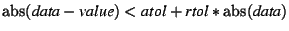(18.1)

That is a careful way of saying that those elements of the data that have a value of value (to within a tolerance) are to be treated as invalid. If data is not of a floating point type, calls masked_object instead.

Creates a masked array with those entries marked invalid that are equal to value. Again, copy and /savespace are passed on to the numarray array constructor.

 asarray( data, type=None)
This is the same as array(data, typecode, copy=0). It is a short way of ensuring that something is an instance of MaskedArray of a given type before proceeding, as in "data = asarray(data)".

If data already is a masked array and type is None then the return value is data; nothing is copied in that case.

Creates a masked array whose shape is that of condition, whose values are those of data, and which is masked where elements of condition are true.

This is a module constant that represents a scalar masked value. For example, if x is a masked array and a particular location such as x is masked, the quantity x will be this special constant. This special element is discussed more fully in section 18.6.1 The constant masked''.

The following additional constructors are provided for convenience.

masked_greater is equivalent to masked_where(greater(data, value), data)). Similarly, masked_greater_equal, masked_equal, masked_not_equal, masked_less, masked_less_equal are called in the same way with the obvious meanings. Note that for floating point data, masked_values is preferable to masked_equal in most cases.

Creates an array with values in the closed interval [v1, v2] masked. v1 and v2 may be in either order.

Creates an array with values outside the closed interval [v1, v2] masked. v1 and v2 may be in either order.

On entry to any of these constructors, data must be any object which the numarray package can accept to create an array (with the desired type, if specified). The mask, if given, must be None or any object that can be turned into a numarray array of integer type (it will be converted to type MaskType, if necessary), have the same shape as data, and contain only values of 0 or 1.

If the mask is not None but its shape does not match that of data, an exception will be thrown, unless one of the two is of length 1, in which case the scalar will be resized (using numarray.resize) to match the other.

See section 18.3.7 Copying or not'' for a discussion of whether or not the resulting array shares its data or its mask with the arguments given to these constructors.

#### Important Tip

filled is very important. It converts its argument to a plain numarray array.

 filled( x, value=None)
Returns x with any invalid locations replaced by a fill value. filled is guaranteed to return a plain numarray array. The argument x does not have to be a masked array or even an array, just something that numarray/numarray.ma can turn into one.
• If x is not a masked array, and not a numarray array, numarray.array(x) is returned.
• If x is a contiguous numarray array then x is returned. (A numarray array is contiguous if its data storage region is layed out in column-major order; numarray allows non-contiguous arrays to exist but they are not allowed in certain operations).
• If x is a masked array, but the mask is None, and x's data array is contiguous, then it is returned. If the data array is not contiguous, a (contiguous) copy of it is returned.
• If x is a masked array with an actual mask, then an array formed by replacing the invalid entries with value, or fill_value(x) if value is None, is returned. If the fill value used is of a different type or precision than x, the result may be of a different type or precision than x.
Note that a new array is created only if necessary to create a correctly filled, contiguous, numarray array.

The function filled plays a central role in our design. It is the exit'' back to numarray, and is used whenever the invalid values must be replaced before an operation. For example, adding two masked arrays a and b is roughly:

masked_array(filled(a, 0) + filled(b, 0), mask_or(getmask(a), getmask(b))

That is, fill the invalid entries of a and b with zeros, add them up, and declare any entry of the result invalid if either a or b was invalid at that spot. The functions getmask and mask_or are discussed later.

filled also can be used to simply be certain that some expression is a contiguous numarray array at little cost. If its argument is a numarray array already, it is returned without copying.

If you are certain that a masked array x contains a mask that is None or is all zeros, you can convert it to a numarray array with the numarray.array(x) constructor. If you turn out to be wrong, an MAError exception is raised.

 fill_value( x)
 fill_value( )
fill_value(x) and the method x.fill_value() on masked arrays, return a value suitable for filling x based on its type. If x is a masked array, then x.fill_value() results. The returned value for a given type can be changed by assigning to the following names in module numarray.ma. They should be set to scalars or one element arrays.
default_real_fill_value = numarray.array([1.0e20], Float32)
default_complex_fill_value = numarray.array([1.0e20 + 0.0j], Complex32)
default_integer_fill_value = numarray.array().astype(UnsignedInt8)

The variable masked is a module variable of numarray.ma and is discussed in section 18.6.1. Calling filled with a fill_value of masked sometimes produces a useful printed representation of a masked array. The function fill_value works on any kind of object.

set_fill_value(a, fill_value) is the same as a.set_fill_value (fill_value) if a is a masked array; otherwise it does nothing. Please note that the fill value is mostly cosmetic; it is used when it is needed to convert the masked array to a plain numarray array but not involved in most operations. In particular, setting the fill_value to 1.e20 will not, repeat not, cause elements of the array whose values are currently 1.e20 to be masked. For that sort of behavior use the masked_value constructor.

Masks are either None or 1-byte numarray arrays of 1's and 0's. To avoid excessive performance penalties, mask arrays are never checked to be sure that the values are 1's and 0's, and supplying a mask argument to a constructor with an illegal mask will have undefined consequences later.

Masks have the savespace attribute set. This attribute, discussed in part I, may have surprising consequences if you attempt to do any operations on them other than those supplied by this package. In particular, do not add or multiply a quantity involving a mask. For example, if m is a mask consisting of 1080 1 values, sum(m) is 56, not 1080. Oops.

Returns true if m is of a type and precision that would be allowed as the mask field of a masked array (that is, it is an array of integers with numarray's typecode MaskType, or it is None). To be a legal mask, m should contain only zeros or ones, but this is not checked.

Returns an object whose entries are equal to m and for which is_mask would return true. If m is already a mask or None, it returns m or a copy of it. Otherwise it will attempt to make a mask, so it will accept any sequence of integers for m. If flag is true, make_mask returns None if its return value otherwise would contain no true elements. To make a legal mask, m should contain only zeros or ones, but this is not checked.

Returns a mask of all zeros of shape s (deprecated name: create_mask).

Returns x.mask(), the mask of x, if x is a masked array, and None otherwise. Note: getmask may return None if x is a masked array but has a mask of None. (Please see caution above about operating on the result).

Returns x.mask() if x is a masked array and has a mask that is not None; otherwise it returns a zero mask array of the same shape as x. Unlike getmask, getmaskarray always returns an numarray array of typecode MaskType. (Please see caution above about operating on the result).

Returns an object which when used as a mask behaves like the element-wise logical or'' of m1 and m2, where m1 and /m2 are either masks or None (e.g., they are the results of calling getmask). A None is treated as everywhere false. If both m1 and m2 are None, it returns None. If just one of them is None, it returns the other. If m1 and m2 refer to the same object, a reference to that object is returned.

## 18.3.6 Operations

Masked arrays support the operators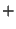,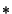,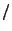,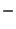,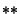, and unary plus and minus. The other operand can be another masked array, a scalar, a numarray array, or something numarray.array can convert to a numarray array. The results are masked arrays.

In addition masked arrays support the in-place operators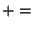,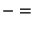,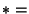, and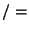. Implementation of in-place operators differs from numarray semantics in being more generous about converting the right-hand side to the required type: any kind or lesser type accepted via an astype conversion. In-place operators truly operate in-place when the target is not masked.

## 18.3.7 Copying or not?

Depending on the arguments results of constructors may or may not contain a separate copy of the data or mask arguments. The easiest way to think about this is as follows: the given field, be it data or a mask, is required to be a numarray array, possibly with a given typecode, and a mask's shape must match that of the data. If the copy argument is zero, and the candidate array otherwise qualifies, a reference will be made instead of a copy. If for any reason the data is unsuitable as is, an attempt will be made to make a copy that is suitable. Should that fail, an exception will be thrown. Thus, a copy=0 argument is more of a hope than a command.

If the basic array constructor is given a masked array as the first argument, its mask, typecode, spacesaver flag, and fill value will be used unless specifically specified by one of the remaining arguments. In particular, if d is a masked array, array(d, copy=0) is d.

Since the default behavior for masks is to use a reference if possible, rather than a copy, which produces a sizeable time and space savings, it is especially important not to modify something you used as a mask argument to a masked array creation routine, if it was a numarray array of typecode MaskType.

## 18.3.8 Behaviors

 float( a)
 int( a)
The conversion operators float, and int are defined to operate on masked arrays consisting of a single unmasked element. Masked values and multi-element arrays are not convertible.
 repr( a)
 str( a)
A masked array defines the conversion operators str and repr by applying the corresponding operator to the numarray array filled(a).

## 18.3.9 Indexing and Slicing

Indexing and slicing differ from Numeric: while generally the same, they return a copy, not a reference, when used in an expression that produces a non-scalar result. Consider this example:

from Numeric import *
x = array([1.,2.,3.])
y = x[1:]
y = 9.
print x

This will print [1., 9., 3.] since x[1:] returns a reference to a portion of x. Doing the same operation using numarray.ma,
from numarray.ma import *
x = array([1.,2.,3.])
y = x[1:]
y = 9.
print x

will print [1., 2., 3.], while y will be a separate array whose present value would be [9., 3.]. While sentiment on the correct semantics here is divided amongst the Numeric Python community as a whole, it is not divided amongst the author's community, on whose behalf this package is written.

## 18.3.10 Indexing in assignments

Using multiple sets of square brackets on the left side of an assignment statement will not produce the desired result:

x = array([[1,2],[3,4]])
x = 20.                           # Error, does not change x
x[1,1] = 20.                            # Correct, changes x

The reason is that x is a copy, so changing it changes that copy, not x. Always use just one single square bracket for assignments.

## 18.3.11 Operations that produce a scalar result

If indexing or another operation on a masked array produces a scalar result, then a scalar value is returned rather than a one-element masked array. This raises the issue of what to return if that result is masked. The answer is that the module constant masked is returned. This constant is discussed in section 18.6.1. While this most frequently occurs from indexing, you can also get such a result from other functions. For example, averaging a 1-D array, all of whom's values are invalid, would result in masked.

## 18.3.12 Assignment to elements and slices

Assignment of a normal value to a single element or slice of a masked array has the effect of clearing the mask in those locations. In this way previously invalid elements become valid. The value being assigned is filled first, so that you are guaranteed that all the elements on the left-hand side are now valid.

Assignment of None to a single element or slice of a masked array has the effect of setting the mask in those locations, and the locations become invalid.

Since these operations change the mask, the result afterwards will no longer share a mask, since masks have copy-on-write semantics.

Send comments to the NumArray community.